Science Courses / Course / Chapter

# Oxidation Number Rules and Examples

Laura Foist, Nissa Garcia
• Author
Laura Foist

Laura has a Masters of Science in Food Science and Human Nutrition and has taught college Science.

• Instructor
Nissa Garcia

Nissa has a masters degree in chemistry and has taught high school science and college level chemistry.

Understand what an oxidation number is and explore the oxidation number rules. Using the rules, solve problems that represent oxidation number examples. Updated: 02/27/2022

Show

## What is an Oxidation Number?

When determining how a reaction will occur or naming compounds, the oxidation number is important. What is an oxidation number? An oxidation number definition is a number assigned to a compound or element to show how many electrons were lost or gained. A negative number shows that electrons were gained, while a positive number shows that electrons were lost. A negative number is used for gaining electrons because electrons have a negative charge, so more electrons will make the compound more negative. If electrons were lost, then the overall result will be more positive, so the oxidation number is positive.

An oxidation number of zero means that the compound or element is in a neutral-free state. For a compound to react with other compounds it needs to share electrons. Oxidation numbers can be assigned to an element, a particular part of a compound, or an entire compound. For a whole compound, the oxidation number is zero as long as it has no ionic charge on it.

## What Is an Oxidation Number?

It's important to know if an atom loses or gains electrons when combining with other atoms to form compounds. How do we keep track of the electrons lost or gained by an atom? We do this by looking at an atom's oxidation number.

An oxidation number is a number that is assigned to an atom in a substance. The oxidation number could be positive, negative, or zero, and it indicates if electrons are lost or gained. In other words, the oxidation number is a number that helps us keep track of electrons in an atom.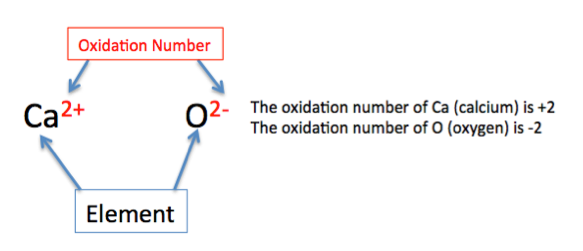Here, the oxidation number of calcium is +2 and the oxidation number of oxygen is -2.

If the oxidation number is positive, then this means that the atom loses electrons, and if it is negative, it means the atom gains electrons. If it is zero, then the atom neither gains nor loses electrons.

Calcium has a +2 charge, which means it lost two electrons. Oxygen has a -2 charge, which means it gained two electrons.

If an atom loses electrons, its oxidation number is positive, so we can say that this atom undergoes oxidation. If an atom gains electrons, its oxidation number is negative, so we can say that the atom undergoes reduction.

You can remember this by thinking of the phrase OIL RIG: Oxidation Is Loss (of electrons); Reduction Is Gain (of electrons).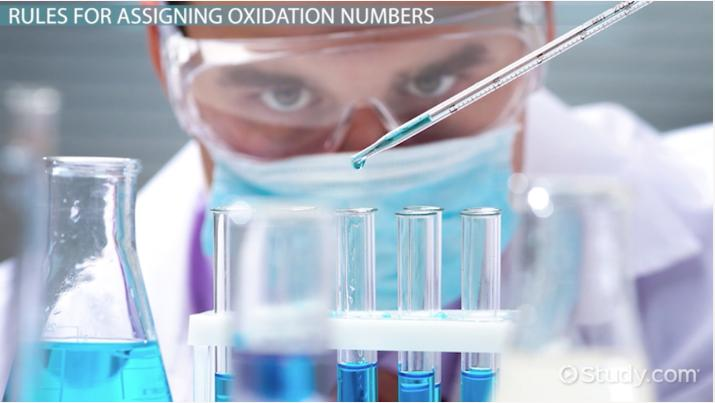An error occurred trying to load this video.

Try refreshing the page, or contact customer support.

Coming up next: What is Xenon? - Definition, Uses & Facts

### You're on a roll. Keep up the good work!

Replay
Your next lesson will play in 10 seconds
• 0:00 What is an Oxidation Number?
• 1:30 Oxidation Number Rules
• 8:30 Lesson Summary
Save Save

Want to watch this again later?

Timeline
Autoplay
Autoplay
Speed Speed

## Assigning Oxidation Numbers Using Rules

When assigning oxidation numbers, specific oxidation number rules need to be followed. These oxidation rules include:

• The oxidation number of a free, neutral element is zero.
• The oxidation number of a monoatomic ion is equal to the charge of the ion.
• The oxidation number of Group IA elements is always +1.
• The oxidation number of Group IIA elements is always +2.
• Oxidation number of oxygen is typically -2.
• Oxidation number of hydrogen is typically +1.
• Oxidation number of Fluorine is ALWAYS -1.
• Oxidation number of other halogens (non-Fluorine) is typically -1 but can vary.
• The total oxidation number of a neutral compound is zero.
• The total oxidation number of elements in a polyatomic ion will sum the charge on the polyatomic ion.

These oxidation rules do not explain the oxidation number for all elements. The oxidation numbers of all other elements (such as carbon or nitrogen) differ based on what they are bound to and can be determined using the above rules. The highest an oxidation number can equal will be the number of valence electrons (the most electrons that the element could lose). The lowest an oxidation number can equal will be 8 (for most elements the most electrons it can hold is 8) minus the number of valence electrons.

## Examples of How to Calculate the Oxidation Number

Finding the oxidation number of elements, ions, and compounds can be determined using the oxidation number rules. The oxidation number examples below show how to find the oxidation number of various species.

### Monatomic Compounds

Monatomic elements are easy to determine the oxidation number because it is equal to the charge seen on the element.

• {eq}LI^+ {/eq}: oxidation number is +1
• {eq}Ba^{2+} {/eq}: oxidation number is +2
• {eq}Fe {/eq}: oxidation number is 0
• {eq}N_2 {/eq}: oxidation number is 0 (even though the nitrogen is bound to another nitrogen, this is the free species of the element nitrogen)
• {eq}Cl^- {/eq}: oxidation number is -1

### Binary Ionic Compounds

The oxidation number of each element in a binary ionic compound is equal to the charge on that element. The charge can be found by looking at the periodic table, and are based on the group that the element is found in:

• Group 1 elements: +1 charge
• Group 2 elements: +2 charge
• Group 13 elements: +3 charge
• Group 14 elements: +4 charge
• Group 15 elements: -3 charge
• Group 16 elements: -2 charge
• Group 17 (halogen) elements: -1 charge
• Group 18 (noble gases) elements: 0 charge (these do not typically react)

The transition metals vary in the charge given. A lot of these charges need to be memorized in order to know the charge given.

The oxidation number of the following ionic compounds can be determined based on the charges:

• {eq}NaCl {/eq}: sodium is in group 1 with an oxidation number of +1, and chlorine is in group 17 with an oxidation number of -1
• {eq}CaI_2 {/eq}: calcium is in group 2, with an oxidation number of +2, iodine is in group 17, with an oxidation number of -1, but there are two of them, so there is an oxidation number of -2
• {eq}Ca_3P_2 {/eq}: calcium is in group 2, with an oxidation number of +2, there are 3 of them, so the total oxidation number is +6. Phosphorous is in group 16, with an oxidation number of +3, there are 2 of them so the total oxidation number is -6
• {eq}FeCl_3 {/eq}: chlorine is in group 17, with an oxidation charge of -1, there are three of them, so the total oxidation number is -3. Iron is a transition metal, and the charge can vary, in this case, it can be determined because the total charge of the compound needs to be 0 since the oxidation number on the chlorine is -3, the oxidation number on the iron needs to be +3.

### Covalent Molecules

To find the oxidation number on covalent molecules it is important to remember that the total oxidation number of a non-charged covalent compound is equal to 0. Start with the elements with specific rules, and work from there, ensuring that the total charge equals 0. Start with any elements that have specific rules, including:

• Oxygen
• Hydrogen
• Halogens
• Group IA elements
• Group IIA elements

Be sure to note important exceptions to each of the rules:

## Rules For Assigning Oxidation Numbers

In chemistry, it is important to follow a set of rules to assign oxidation numbers. Here are the most important rules and exceptions to remember when assigning oxidation numbers:

Rule 1: In its pure elemental form, an atom has an oxidation number of zero

What exactly is a pure element? A pure element is an atom that is not combined with any other elements. This table shows examples of atoms in their pure elemental form: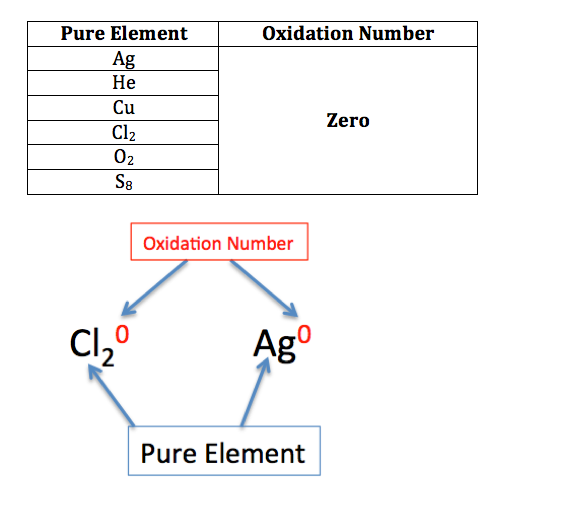Take note that even though the element has subscripts like the 2 in O2 and the 8 in S8, these are still considered pure elements because they are not combined with any other elements.

Rule 2: The oxidation number of an ion is the same as its charge

An ion is an atom with a charge due to the gain or loss of electrons. The charge is indicated as the superscript after the atom. If an atom has this, then this means that it is an ion. For example, the potassium ion has a +1 charge, so the oxidation number is +1. For the bromine ion, the charge is -1, so the oxidation number is -1. It is important to note that if only a positive (+) or negative (-) is shown, the charge is assumed to be +1 or -1.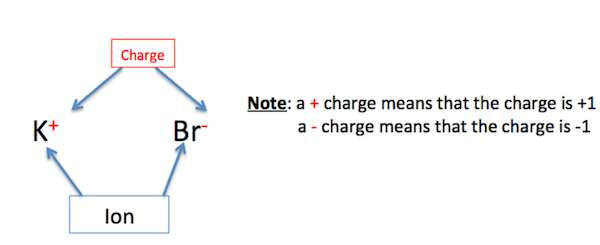Here is another example. The barium ion has a +2 charge, so the oxidation number is +2. For the sulfur ion, the charge is -2, and the oxidation number is -2.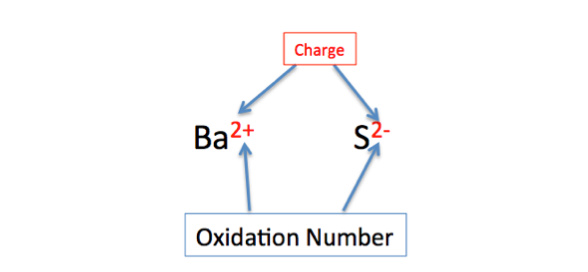Rule 3: The oxidation number of metals is +1 in Group 1 and +2 in Group 2

Unless it is in pure elemental form, the oxidation number of a metal is +1 in Group 1 and +2 in Group 2. It is important to note that although hydrogen is in Group 1, hydrogen is not a metal, so hydrogen is not included in this rule. In the following illustration, the metals for Group 1 and 2 are indicated.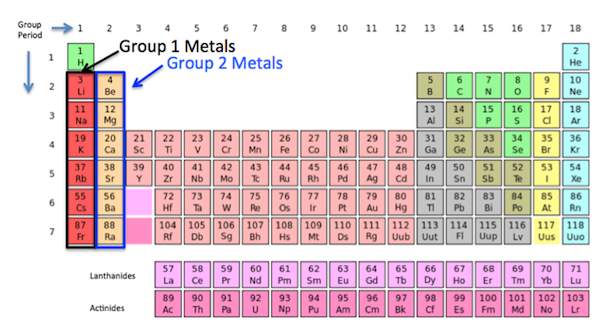So, the oxidation number for lithium is +1 because it is a metal that belongs in Group 1, and the oxidation number of magnesium is +2 because it is a metal that belongs in Group 2.

Rule 4: Hydrogen has two possible oxidation numbers: +1 and -1

Hydrogen has an oxidation number of +1 when it is bonded to nonmetals, which are highlighted on the right side of the following periodic table.

For the compound hydrochloric acid, hydrogen is bonded to chlorine, a nonmetal, so the oxidation number of hydrogen is +1.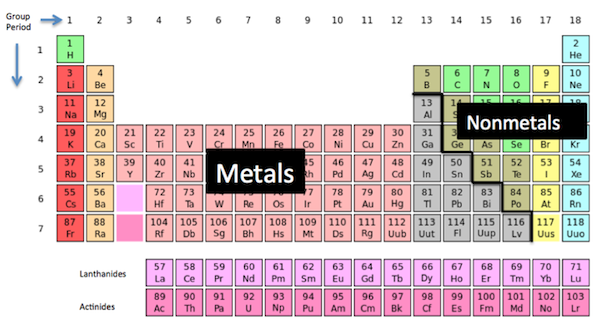Hydrogen has an oxidation number of -1 when it is bonded to a metal.

For the compound sodium hydride, hydrogen is bonded to sodium, which is a metal, so the oxidation number of hydrogen is -1.

Rule 5: Oxygen has three possible oxidation numbers: +2, -2 and -1

In general, oxygen has an oxidation number of -2. The only exceptions are peroxides, where oxygen has an oxidation number of -1, and in the compound of oxygen difluoride, where it has an oxidation number of +2.

Rule 6: The oxidation number of fluorine in any compound is -1

A few examples of fluorine-containing compounds are hydrogen fluoride or hydrofluoric acid, sulfur hexafluoride, and sodium fluoride. The oxidation number of fluorine in each of these compounds is -1.

Rule 7: For the other halogens (Cl, Br, I), the usual oxidation number is -1, except when they are combined with oxygen or fluorine.

In the compound sodium chloride, the oxidation number of chlorine is -1. In the compound hypochlorous acid, the oxidation number of chlorine is +1; and in the compound perchloric acid, the oxidation number of chlorine is +7.

Rule 8: The sum of the oxidation numbers in a neutral compound is equal to zero

Here are a few examples of neutral compounds: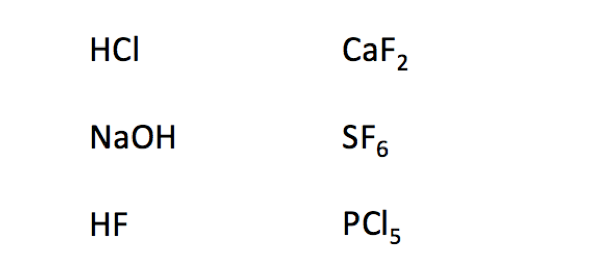Let's take a look at two examples to see how to calculate the oxidation numbers of the elements in a neutral compound using the rules we have learned so far.

Example 1:

Here we have the molecule hydrogen fluoride, which is a neutral compound, so its oxidation number is 0. To determine the oxidation numbers of the elements hydrogen and fluorine in this compound, we multiply the number of hydrogen atoms by the oxidation number of hydrogen. Then, multiply the number of fluorine atoms by the oxidation number of fluorine.

Hydrogen has an oxidation number of +1, and we have 1 hydrogen atom:

1 * (1) = 1

Fluorine has an oxidation number of -1, and we have 1 fluorine atom:

1 * (-1) = -1

(-1) + 1 = 0

Example 2:

To unlock this lesson you must be a Study.com Member.

Video Transcript

## What Is an Oxidation Number?

It's important to know if an atom loses or gains electrons when combining with other atoms to form compounds. How do we keep track of the electrons lost or gained by an atom? We do this by looking at an atom's oxidation number.

An oxidation number is a number that is assigned to an atom in a substance. The oxidation number could be positive, negative, or zero, and it indicates if electrons are lost or gained. In other words, the oxidation number is a number that helps us keep track of electrons in an atom.Here, the oxidation number of calcium is +2 and the oxidation number of oxygen is -2.

If the oxidation number is positive, then this means that the atom loses electrons, and if it is negative, it means the atom gains electrons. If it is zero, then the atom neither gains nor loses electrons.

Calcium has a +2 charge, which means it lost two electrons. Oxygen has a -2 charge, which means it gained two electrons.

If an atom loses electrons, its oxidation number is positive, so we can say that this atom undergoes oxidation. If an atom gains electrons, its oxidation number is negative, so we can say that the atom undergoes reduction.

You can remember this by thinking of the phrase OIL RIG: Oxidation Is Loss (of electrons); Reduction Is Gain (of electrons).

## Rules For Assigning Oxidation Numbers

In chemistry, it is important to follow a set of rules to assign oxidation numbers. Here are the most important rules and exceptions to remember when assigning oxidation numbers:

Rule 1: In its pure elemental form, an atom has an oxidation number of zero

What exactly is a pure element? A pure element is an atom that is not combined with any other elements. This table shows examples of atoms in their pure elemental form:Take note that even though the element has subscripts like the 2 in O2 and the 8 in S8, these are still considered pure elements because they are not combined with any other elements.

Rule 2: The oxidation number of an ion is the same as its charge

An ion is an atom with a charge due to the gain or loss of electrons. The charge is indicated as the superscript after the atom. If an atom has this, then this means that it is an ion. For example, the potassium ion has a +1 charge, so the oxidation number is +1. For the bromine ion, the charge is -1, so the oxidation number is -1. It is important to note that if only a positive (+) or negative (-) is shown, the charge is assumed to be +1 or -1.Here is another example. The barium ion has a +2 charge, so the oxidation number is +2. For the sulfur ion, the charge is -2, and the oxidation number is -2.Rule 3: The oxidation number of metals is +1 in Group 1 and +2 in Group 2

Unless it is in pure elemental form, the oxidation number of a metal is +1 in Group 1 and +2 in Group 2. It is important to note that although hydrogen is in Group 1, hydrogen is not a metal, so hydrogen is not included in this rule. In the following illustration, the metals for Group 1 and 2 are indicated.So, the oxidation number for lithium is +1 because it is a metal that belongs in Group 1, and the oxidation number of magnesium is +2 because it is a metal that belongs in Group 2.

Rule 4: Hydrogen has two possible oxidation numbers: +1 and -1

Hydrogen has an oxidation number of +1 when it is bonded to nonmetals, which are highlighted on the right side of the following periodic table.

For the compound hydrochloric acid, hydrogen is bonded to chlorine, a nonmetal, so the oxidation number of hydrogen is +1.Hydrogen has an oxidation number of -1 when it is bonded to a metal.

For the compound sodium hydride, hydrogen is bonded to sodium, which is a metal, so the oxidation number of hydrogen is -1.

Rule 5: Oxygen has three possible oxidation numbers: +2, -2 and -1

In general, oxygen has an oxidation number of -2. The only exceptions are peroxides, where oxygen has an oxidation number of -1, and in the compound of oxygen difluoride, where it has an oxidation number of +2.

Rule 6: The oxidation number of fluorine in any compound is -1

A few examples of fluorine-containing compounds are hydrogen fluoride or hydrofluoric acid, sulfur hexafluoride, and sodium fluoride. The oxidation number of fluorine in each of these compounds is -1.

Rule 7: For the other halogens (Cl, Br, I), the usual oxidation number is -1, except when they are combined with oxygen or fluorine.

In the compound sodium chloride, the oxidation number of chlorine is -1. In the compound hypochlorous acid, the oxidation number of chlorine is +1; and in the compound perchloric acid, the oxidation number of chlorine is +7.

Rule 8: The sum of the oxidation numbers in a neutral compound is equal to zero

Here are a few examples of neutral compounds:Let's take a look at two examples to see how to calculate the oxidation numbers of the elements in a neutral compound using the rules we have learned so far.

Example 1:

Here we have the molecule hydrogen fluoride, which is a neutral compound, so its oxidation number is 0. To determine the oxidation numbers of the elements hydrogen and fluorine in this compound, we multiply the number of hydrogen atoms by the oxidation number of hydrogen. Then, multiply the number of fluorine atoms by the oxidation number of fluorine.

Hydrogen has an oxidation number of +1, and we have 1 hydrogen atom:

1 * (1) = 1

Fluorine has an oxidation number of -1, and we have 1 fluorine atom:

1 * (-1) = -1

(-1) + 1 = 0

Example 2:

To unlock this lesson you must be a Study.com Member.

#### What is the oxidation number in chemistry?

The oxidation number is a number assigned to elements to show how many electrons were lost or gained. A positive number means electrons were lost. A negative number means electrons were gained.

#### What are oxidation number rules?

The oxidation number rules are rules for finding the oxidation number of elements. These include: the sum of each element oxidation number needs to total the charge on the compound and the oxidation number is often equal to the charge on the element, unless bound to a more electronegative element.

#### How do you find the oxidation number?

The oxidation number is often found by knowing the charge on the element. Exceptions occur when the element is bound to a more electronegative element.

### Register to view this lesson

Are you a student or a teacher?

Back

### Resources created by teachers for teachers

Over 30,000 video lessons & teaching resources‐all in one place.Video lessonsQuizzes & WorksheetsClassroom IntegrationLesson Plans

I would definitely recommend Study.com to my colleagues. It’s like a teacher waved a magic wand and did the work for me. I feel like it’s a lifeline.

Jennifer B.
TeacherCreate an account to start this course today
Used by over 30 million students worldwide